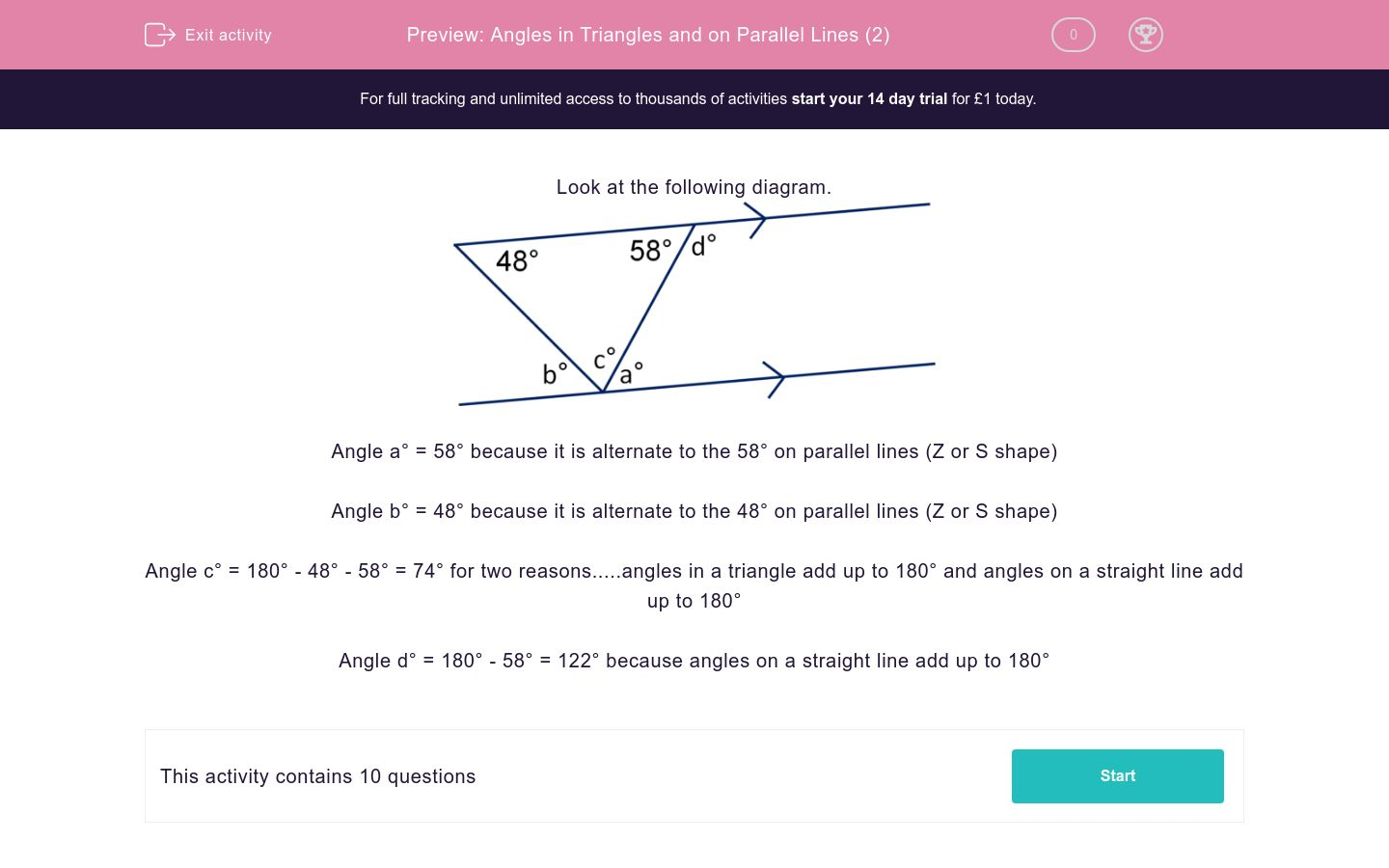# Angles in Triangles and on Parallel Lines (2)

In this worksheet, students use angle rules to work out the missing angles in triangles and parallel lines.Key stage:  KS 2

Curriculum topic:   Maths and Numerical Reasoning

Curriculum subtopic:   2D Shapes: Triangles, Quadrilaterals and Polygons

Difficulty level:### QUESTION 1 of 10

Look at the following diagram.Angle a° = 58° because it is alternate to the 58° on parallel lines (Z or S shape)

Angle b° = 48° because it is alternate to the 48° on parallel lines (Z or S shape)

Angle c° = 180° - 48° - 58° = 74° for two reasons.....angles in a triangle add up to 180° and angles on a straight line add up to 180°

Angle d° = 180° - 58° = 122° because angles on a straight line add up to 180°

Look at the following diagram and work out the value of a.

(Just write the number)Look at the following diagram and work out the value of c.

(Just write the number)Look at the following diagram and work out the value of d.

(Just write the number)Look at the following diagram and work out the value of a.

(Just write the number)Look at the following diagram and work out the value of b.

(Just write the number)Look at the following diagram and work out the value of c.

(Just write the number)Look at the following diagram and work out the value of d.

(Just write the number)Look at the following diagram and work out the value of e.

(Just write the number)Look at the following diagram and work out the value of f.

(Just write the number)Look at the following diagram and work out the value of g.

(Just write the number)• Question 1

Look at the following diagram and work out the value of a.

(Just write the number)55
EDDIE SAYS
a is an alternate (Z) angle to 55°
• Question 2

Look at the following diagram and work out the value of c.

(Just write the number)57
EDDIE SAYS
c is on a striaght line with 123° c = 180 - 123
• Question 3

Look at the following diagram and work out the value of d.

(Just write the number)68
EDDIE SAYS
We have previously worked out that; a = 55° c = 57° d is in a triangle with a and c so all three must add up to 180° d = 180 - 55 - 57
• Question 4

Look at the following diagram and work out the value of a.

(Just write the number)45
EDDIE SAYS
a is alternate to 45°
• Question 5

Look at the following diagram and work out the value of b.

(Just write the number)73
EDDIE SAYS
b is on a straight line with 107° b = 180 - 107
• Question 6

Look at the following diagram and work out the value of c.

(Just write the number)73
EDDIE SAYS
Two reasons here. 1) C is alternate to b and we have just found that b = 73 2) C is co-interior/supplementary to 107° 180 - 107
• Question 7

Look at the following diagram and work out the value of d.

(Just write the number)62
EDDIE SAYS
We have previously worked out that b = 73 b. d and 45 are in a triangle and must add up to 180 180 - 45 - 73
• Question 8

Look at the following diagram and work out the value of e.

(Just write the number)135
EDDIE SAYS
We previously found that a = 45 a and e are on a straight line 180 - 45
• Question 9

Look at the following diagram and work out the value of f.

(Just write the number)45
EDDIE SAYS
Two reasons again. 1) We just worked out that e = 135° and e and f are on a straight line so 180 - 135 2) a and f are vertically opposite angles (which are equal)
• Question 10

Look at the following diagram and work out the value of g.

(Just write the number)73
EDDIE SAYS
We worked out that b = 73 g is vertically opposite to b and must therefore be equal.
---- OR ----